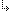- 6.10. -6. Win32 Programming6.10. Using Dinamic-Link Libraries (DLLs)

## 6.10. Using Dinamic-Link Libraries (DLLs)

Targets: OS/2, Win32

TMT Pascal provides three ways to import procedures and functions:

By new name:
When a procedure or function is imported with a name clause specified, it is imported by a different name than its identifier.

By index:
When a procedure or function is imported with an index clause specified, it is imported by an ordinal (i.e. a given index).

By name:
When a procedure or function is imported with no index or name clause specified, it is imported explicitly by it's identifier name.

Example:

This external declaration imports the function ExitProcess from the system DLL called KERNEL32 (the Windows 32 kernel):
```procedure ExitProcess conv arg_stdcall (uExitCode: DWORD);
external kernel32dll name 'ExitProcess';```
Example:
This example program imports ArcCos and ArcSin functions from the DLL called ARCs (see Writing DLLs):
```program TestDLL;
uses
Strings;

const
ARCs = 'arcs.dll';

// import by name
{\$ifdef __WIN32__}
function ArcCos conv arg_stdcall (X: Extended): Extended;
external ARCs name 'ArcCos';
{\$else}
function ArcCos conv arg_os2 (X: Extended): Extended;
external ARCs name 'ArcCos';
{\$endif}

// import by index
{\$ifdef __WIN32__}
function ArcSin conv arg_stdcall (X: Extended): Extended;
external ARCs index 1;
{ \$else}
function ArcSin conv arg_os2 (X: Extended): Extended;
external ARCs index 1;
{\$endif}

var Arg: Extended;

begin
repeat
Write('Argument ? ');